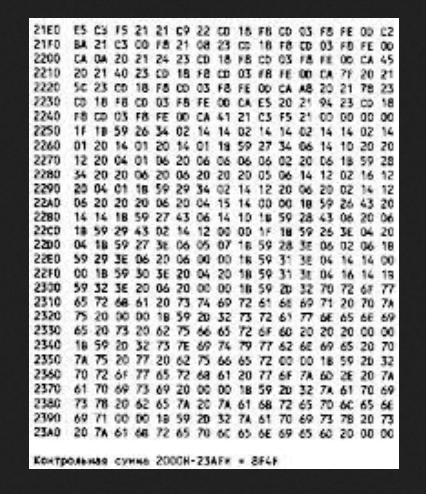## [Math] Remainder as a checksum

As seen in Wikipedia:

A very practical application is to calculate checksums within serial number identifiers. For example, International Standard Book Number (ISBN) uses modulo 11 (for 10 digit ISBN) or modulo 10 (for 13 digit ISBN) arithmetic for error detection. Likewise, International Bank Account Numbers (IBANs), for example, make use of modulo 97 arithmetic to spot user input errors in bank account numbers. In chemistry, the last digit of the CAS registry number (a unique identifying number for each chemical compound) is a check digit, which is calculated by taking the last digit of the first two parts of the CAS registry number times 1, the previous digit times 2, the previous digit times 3 etc., adding all these up and computing the sum modulo 10.

( Wikipedia: Modular_arithmetic#Applications )

Oh yes, it can be used as a checksum.

Some of us who are from 1980s times, may remember type-in programs. The same was in USSR era. Programs (usually, written in assembly language) for 8-bit home computers were printed in electronics magazines in a hexadecimal form. (Pardon blurry screenshot.)The last line is the checksum of the whole dump.

Now the problem. If the checksum is calculated as a simple sum, it will not be very reliable. Add one bit to one byte and subtract from another byte:

Python 3.8.10 (default, Nov 26 2021, 20:14:08)
[GCC 9.3.0] on linux
>>> dump=[0x11, 0x78, 0xab, 0x10]
>>> sum(dump)
324
>>> dump2=[0x10, 0x78, 0xab, 0x11]
>>> sum(dump2)
324


That method can't catch such (two) typos or bugs.

The whole buffer (no matter how long) can be represented as a (huge) number.

(This example only for 4-byte buffers, but can be extended, of course.)

>>> struct.unpack("i", bytearray(dump))
279672849
>>> struct.unpack("i", bytearray(dump2))
296450064


Pick a prime that is close to 0xFFFF: 65521 (or 0xFFF1). Now divide these numbers by this prime and get a remainder:

>>> struct.unpack("i", bytearray(dump2)) % 65521
33060
>>> struct.unpack("i", bytearray(dump)) % 65521
29221


>>> "%x" % (struct.unpack("i", bytearray(dump)) % 65521)
'7225'
>>> "%x" % (struct.unpack("i", bytearray(dump2)) % 65521)
'8124'


The remainder is very sensitive to bit flips.

Also, you can't use divisors like 0x100 or 0x10000. The first will take into account only last 8 bits. The second -- only last 16 bits.

But a prime as a divisor will 'process' all input bits, no matter how big the number/buffer/file is.

Now the CRC. In fact, CRC does the same -- it 'divides' the input 'huge number' by a GF(2) polynomial, but not in a conventional arithmetic. Rather in GF(2). Such an esoteric math is used because GF(2) divisor is way easier to implement in hardware. While an usual divisor includes adder or subtractor, which has carry-ripple (in simple cases), and very cumbersome, in comparison with GF(2) divisor.

Also, some people say that CRC polynomials should be irreducible. This is analog to prime numbers. (However, some other say that this is not a strict requirement.)